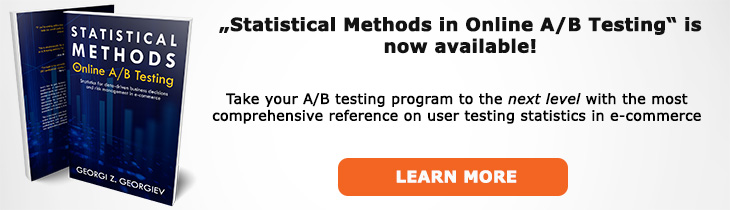# What does "Absolute Difference" mean?

Definition of Absolute Difference in the context of A/B testing (online controlled experiments).

## What is Absolute Difference?

Aliases: absolute change

The absolute difference between two variables, for example two means or proportions is simply one subtracted from the other. It is often written as δB,A or δabs = μ1 - μ2, equivalently δabs = p1 - p2 for proportions. For example, if the proportion in group B is 0.05 and the proportion in group A is 0.04, then δB,A = 0.05 - 0.04 = 0.01.

It should be noted that most traditional significance tests and confidence intervals are developed to address the question in terms of absolute difference which is why they are convenient when this is the primary parameter of interest. However, one cannot make a naive extrapolation from this to relative difference / percentage change since calculating such statistics needs to take into account the increased variance due to the division by the control group conversion rate. The differences are especially large when the actual difference is large.

Special methods are developed to construct CIs, calculate p-values and perform sample size calculations when one is interested in making claims about relative change (% lift), see percent change for more on this.

Percent Change

## Articles on Absolute Difference

Like this glossary entry? For an in-depth and comprehensive reading on A/B testing stats, check out the book "Statistical Methods in Online A/B Testing" by the author of this glossary, Georgi Georgiev.## Glossary Index by Letter

Select a letter to see all A/B testing terms starting with that letter or visit the Glossary homepage to see all.